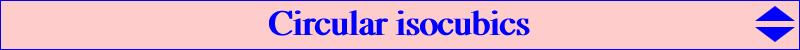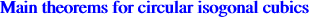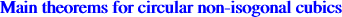A cubic is said to be circular when it contains the two circular points at infinity (which are isogonal conjugates) and therefore another real point at infinity. The tangents at the circular points at infinity intersect at a point called singular focus of the cubic. When this focus lies on the curve, the cubic is said to be a (van Rees) focal cubic. The most remarkable circular cubic is the Neuberg cubic which is an isogonal circular pK. The Droussent cubic is the only isotomic circular pK. A study of these cubics can be found in Special Isocubics §4 where many examples are provided. See also table 12 and CL035, the class of circular pKs. Here is a short summary of the results found in this §4 :Theorem 1 : all isogonal circular pKs form a pencil of cubics with a pivot on the line at infinity. They all contain A, B, C, I, Ia, Ib, Ic and the two circular points at infinity. The singular focus is the antipode on the circumcircle of the isogonal conjugate of the real point at infinity of the cubic. This pencil contains only three focal cubics obtained when the pivot is the infinite point of an altitude. The cubic decomposes into a circle and a line if and only if the pivot is the infinite point of a bisector. Theorem 2 : all isogonal circular nK form a net of cubics. They all are focal cubics with singular focus on the circumcircle.Denote by W (different of K) the pole of the isoconjugation. Theorem 3 : for a given W, there is only one non-isogonal circular pK. Theorem 4 : for a given W, all circular nKs form a pencil of cubics. This pencil contains only one focal cubic.